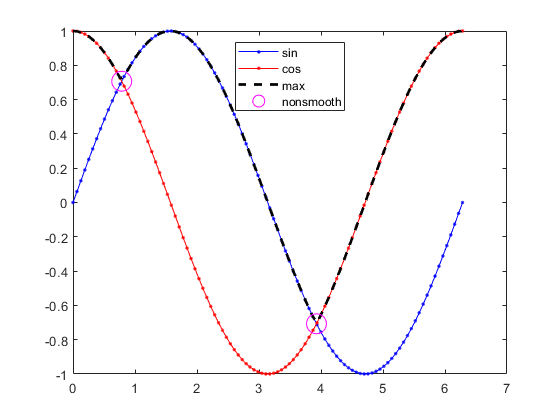## Smooth Formulations of Nonsmooth Functions

To smooth an otherwise nonsmooth problem, you can sometimes add auxiliary variables. For example,

f(x) = max(g(x),h(x))

can be a nonsmooth function even when g(x) and h(x) are smooth, as illustrated by the following functions.

`$\begin{array}{l}g\left(x\right)=\mathrm{sin}\left(x\right)\\ h\left(x\right)=\mathrm{cos}\left(x\right)\\ f\left(x\right)=\mathrm{max}\left(g\left(x\right),h\left(x\right)\right).\end{array}$`

f(x) is nonsmooth at the points x = π/4 and x = 5π/4.This lack of smoothness can cause problems for Optimization Toolbox™ solvers, all of which assume that objective functions and nonlinear constraint functions are continuously differentiable. So, if you try to solve

x = mint(f(t)) starting from the point `x0` = 1,

you do not get an exit flag of 1, because the solution is not differentiable at the locally minimizing point x = π/4.

```fun1 = @sin; fun2 = @cos; fun = @(x)max(fun1(x),fun2(x)); [x1,fval1,eflag1] = fminunc(fun,1)```
```Local minimum possible. fminunc stopped because it cannot decrease the objective function along the current search direction. <stopping criteria details> x1 = 0.7854 fval1 = 0.7071 eflag1 = 5```

Sometimes, you can use an auxiliary variable to turn a nonsmooth problem into a smooth problem. For the previous example, consider the auxiliary variable y with the smooth constraints

`$\begin{array}{l}y\ge g\left(x\right)\\ y\ge h\left(x\right).\end{array}$`

Consider the optimization problem, subject to these constraints,

`$\underset{x}{\mathrm{min}}y.$`

The resulting solution `x`, `y` is the solution to the original problem

`$\underset{x}{\mathrm{min}}f\left(x\right)=\underset{x}{\mathrm{min}}\mathrm{max}\left(g\left(x\right),h\left(x\right)\right).$`

This formulation uses the problem-based approach.

```myvar = optimvar("myvar"); auxvar = optimvar("auxvar"); smprob = optimproblem("Objective",auxvar); smprob.Constraints.cons1 = auxvar >= sin(myvar); smprob.Constraints.cons2 = auxvar >= cos(myvar); x0.myvar = 1; x0.auxvar = 1; [sol2,fval2,eflag2] = solve(smprob,x0)```
```Solving problem using fmincon. Local minimum found that satisfies the constraints. Optimization completed because the objective function is non-decreasing in feasible directions, to within the value of the optimality tolerance, and constraints are satisfied to within the value of the constraint tolerance. sol2 = struct with fields: auxvar: 0.7071 myvar: 0.7854 fval2 = 0.7071 eflag2 = OptimalSolution```

This same concept underlies the formulation of the `fminimax` function; see Goal Attainment Method.